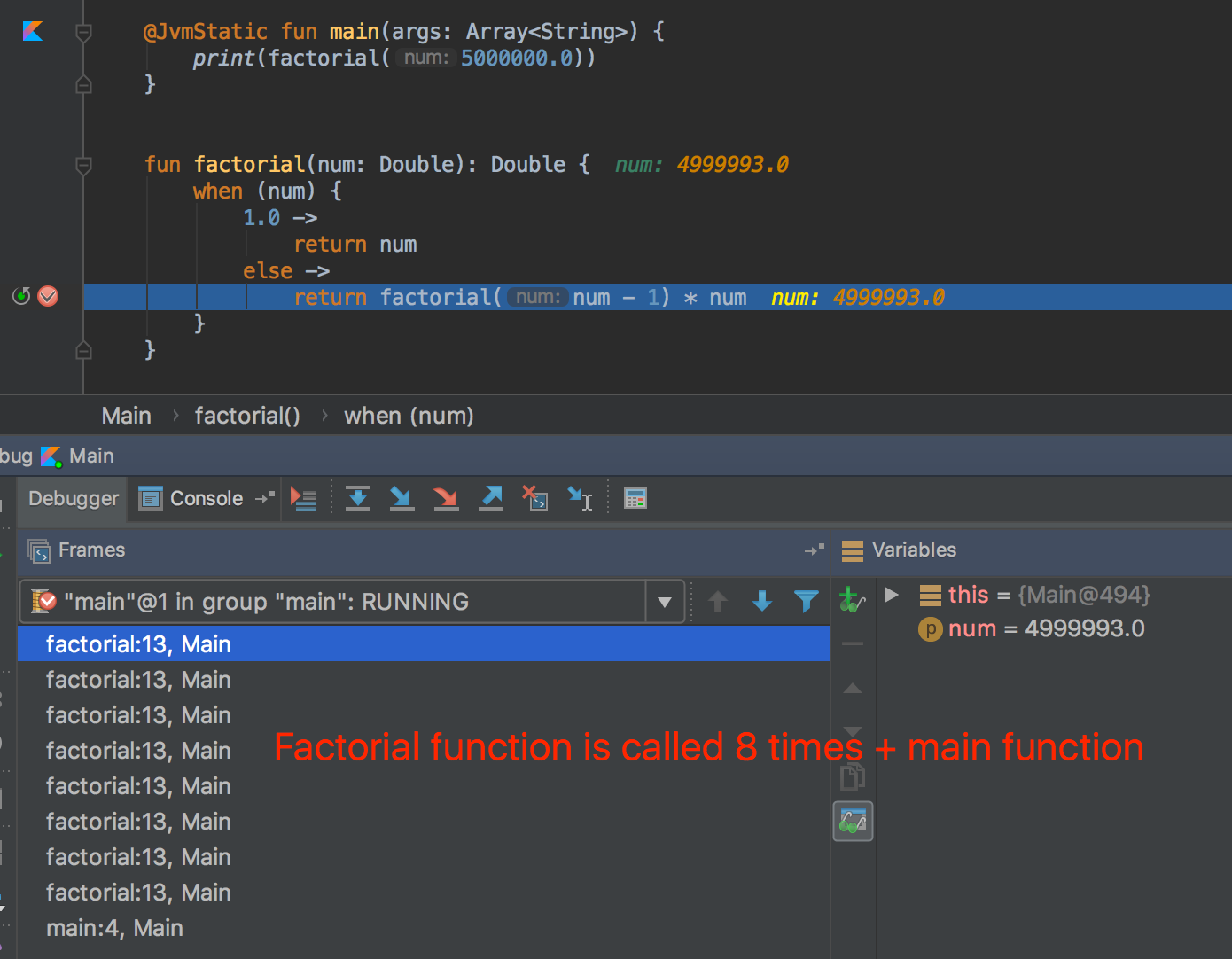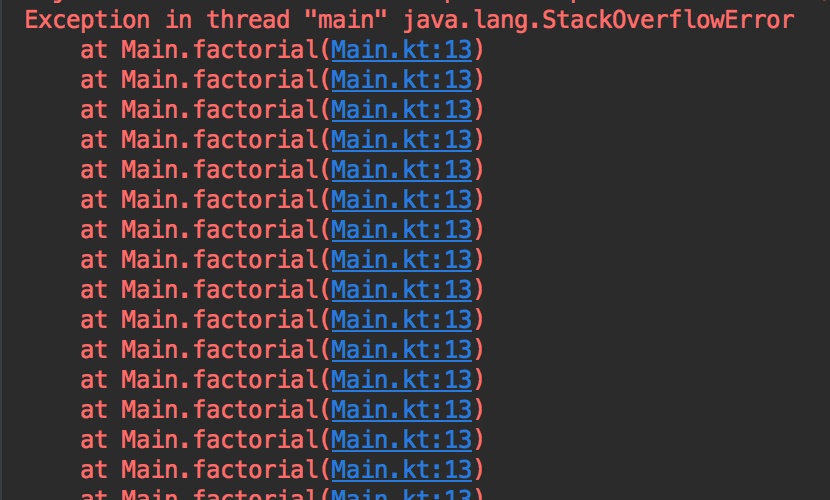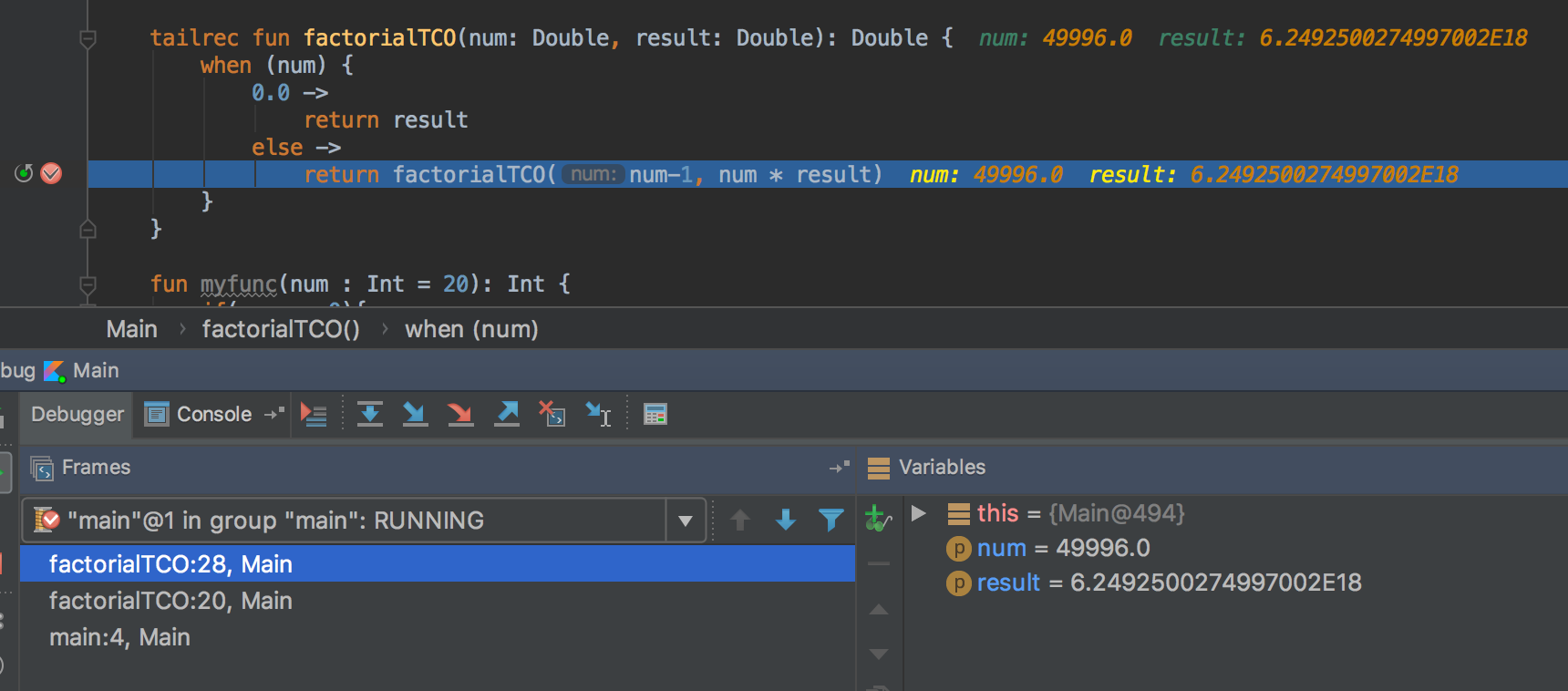# Tail Call Optimization in Kotlin

How to make use of recursion in Kotlin.

Since Kotlin is a multi-paradigm language, we can also avoid using for loop and use recursion instead. This blog, I will show a little trick to do a TCO in Kotlin.

The Imperative Programing is normally defined by thing four things:
Sequence, Selection, Iteration, Subroutine

But in the FP, it has no Sequence and Iteration concepts. So thinking about when we have no loop to use in our program, it seems that it has only a recursive function which still we can use.
Let me writing a short recursive function which will find a factorial result:

``````fun factorial(num: Double): Double {
when (num) {
1.0 ->
return num
else ->
return factorial(num - 1) * num
}
}
``````

This code is working fine. It can give you an expected result but what if I tell you that it could cause you a problem?

StackOverflow ErrorFactorial function is called 8 times + main function

Every time when we call a function, one stack frame will be created. Let’s say that I call this function 50,000 times which will create 50,000 frames in stack and when it reach the heap size then this error will happen. Moreover, in term of the performance, this code will also give you a bad result.StackOverflow Error when function is called multiple times

Tail call optimization
To solve the problem, there is the way we can do to our code to a tail recursion which that means in the line that function call itself must be the last line and it must not have any calculation after it. For example:

``````fun myfunc(num : Int = 20): Int {
if(num == 0){
return 0
} else {
return myfunc()
}
}
``````

The above function is a tail recursion function. Here is another sample which is NOT a tail recursion.

``````fun notaTailRecursionFunction(num : Int = 20): Int {
if(num == 0){
return 0
} else {
return notaTailRecursionFunction() + 10
}
}
``````

The function above, even it return itself at the last line but it still does some calculate ( + 10) which isn’t correct.

Let’s optimize the factorial function:

``````private fun factorial(num: Double): Double {
return factorial(num, 1.0)
}

fun factorial(num: Double, result: Double): Double {
when (num) {
0.0 ->
return result
else ->
return factorial(num-1, num * result)
}
}
``````

I simply split it as two functions and make sure both of them follow the tail recursion concept.

Is that all?
No, same as Scala @tailrec, in Kotlin will still need to define a tail recursion function which a tailrec syntax, unless it still give you the same problem.

``````private fun factorial(num: Double): Double {
return factorial(num, 1.0)
}

tailrec fun factorial(num: Double, result: Double): Double {
when (num) {
0.0 ->
return result
else ->
return factorial(num-1, num * result)
}
}
``````

Let’s run the program and see the stack frame:Only 3 frames are shown in the stack

So every time you like to do a recursion, 2 things that need to consider which are:
1. Make the function as tail recursion
2. Put tailrec in front of your function.

If your function isn’t a tail call but you put tailrec in front of it, the Jetbrain IDE is smart enough to tell you that it isn’t. :)